Warning: Illegal string offset 'html' in /home/hsn/public_html/forum/cache/skin_cache/cacheid_1/skin_topic.php on line 909

trigonometry question - HSN forum# trigonometry question

12 replies to this topic

### #1naav

• Gender:Male

Posted 24 September 2006 - 10:14 PM

Hi everyone.Could anyone tell me where I've gone wrong in my solution to the following question.

----------------------------------

The Water Board of a local authority discovered it was able to represent the approximate amount of water W(t), in millions of gallons, stored in a reservoir t months after 1st May 1988 by the formula W(t) = 1.1 - sin[(pi)(t)/6]

The board then predicted that under normal conditions this formula would apply for three years.

On Ist April 1990 a serious fire required an extra 1/4 million gallons of water from the reservoir to bring the fire under control.
Assuming that the previous trend continues from the new lower level, when will the reservoir run dry if water rationing is not imposed?

-----------------------------------

okay for 1.1 - sin[(pi)(t)/6], I let t = 23...as the number of months between 1 May 1988 and 1 April 1990 is 23 months...

I then got the amount of water to be 1.605...

I then said that 1.605 - sin[(pi)(t)/6] - 0.25 = 0...

then sin[(pi)(t)/6] = 1.355...

but I can't get the inverse sin of 1.355 as it comes up as 'error'...???...

-----------------------------------

Thank you.### #2Steve

Top of the Class

••• 435 posts
• Location:Edinburgh
• Gender:Male

Posted 25 September 2006 - 03:50 PM

Are you sure the amount needed for the fire is 1/4 million gallons? I makes sense that you'd get an error for this since sine is always between -1 and 1 (inclusive).
HSN contribute: Help the site grow!

Looking for a Maths tutor in West Lothian? Just PM me!

### #3naav

• Gender:Male

Posted 25 September 2006 - 08:57 PM

Hi, thank you.

The question says the amount needed for the fire is 1/4 million gallons so I think I'm sure### #4George

Child Prodigy

••• 720 posts
• Location:West Lothian
• Gender:Male

Posted 25 September 2006 - 09:48 PM

OK, I think I've got it.

You don't actually need to work out W(23) - the question is really asking "Find the first value of t>23 for which W(t)-0.25=0"

Here's a graph of W(t) [upper] and W(t)-0.25 [lower]

[attachmentid=204]

So the reservoir follows the top wave until t=23, when it suddenly jumps down to the lower wave (since the water has been taken out for the fire).

As you can see, that hits zero at about t=26 - corresponding to the reservoir running dry.

You can't just use a picture though - you'll need to solve the equation### #5naav

• Gender:Male

Posted 25 September 2006 - 10:07 PM

Hi, thank you very much.

Okay, howz this...

1.1 - sin[(pi)(t)/6] - 0.25

sin[(pi)(t)/6] = 0.85

then since sin is positive in the 1st and 2nd quadrants...

[(pi)(t)/6] = 1.016 or 3.14 - 1.016

then

t = 1.94 or 4.06

I then wrote that 1 month = 30/31 days so 1.94 days = 58.2/60.14 days

so

1st April + 58.2/60.14 days = 28th may/30th May

-------------------------------------------
However the textbook gives the answer as 28th June...???...

### #6George

Child Prodigy

••• 720 posts
• Location:West Lothian
• Gender:Male

Posted 26 September 2006 - 09:15 AM

This is quite tricky, and I've only got a couple of minutes to write this postYou started off OK, but the main point here is that you are looking for solutions outside the usual range. You can see from the lower graph that there are 4 roots where t<23, but we aren't interested in those.

So we have: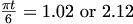We also know there are additional solutions every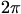, so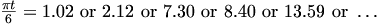Going by the graph, it's that last one (the 5th root along) that should be our solution.

Indeed, using that we get t=25.95. This is 2 years and 1.95 months after 1st May 1988, which I think works out at about 28th June.

Has that helped?

### #7naav

• Gender:Male

Posted 26 September 2006 - 10:03 PM

Hi, thank you very muchYes, that has helped!

Could I please ask why in my first post I got my solution wrong - how come it didn't work out?

Thank you### #8George

Child Prodigy

••• 720 posts
• Location:West Lothian
• Gender:Male

Posted 27 September 2006 - 12:23 PM

okay for 1.1 - sin[(pi)(t)/6], I let t = 23...as the number of months between 1 May 1988 and 1 April 1990 is 23 months...

I then got the amount of water to be 1.605...

I then said that 1.605 - sin[(pi)(t)/6] - 0.25 = 0...

then sin[(pi)(t)/6] = 1.355...

but I can't get the inverse sin of 1.355 as it comes up as 'error'...???...

I've highlighted the bit where I think it goes wrong. There's no reason to change the 1.1 to a 1.605 there.

You got it right later on though, when you were solving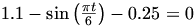. The twist with this question is that you want the first solution where t>23, which is outside the normal 0 to 2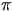range we're used to.

### #9naav

• Gender:Male

Posted 27 September 2006 - 09:11 PM

Hi, thank you very much.But doesn't the 1.605 represent the amount of water after 23 months as I put in t = 23 to find out that amount...and then I took away the amount needed for the fire i.e. 0.25...it's strange how the equation doesn't work out after that...I just wondered why that is...

### #10George

Child Prodigy

••• 720 posts
• Location:West Lothian
• Gender:Male

Posted 27 September 2006 - 10:12 PM

OK, here's a plot of the three curves we're talking about:

[attachmentid=205]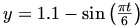(red) - the curve we start with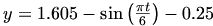(green) - the curve you tried first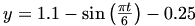(yellow) - the curve we need to use

From that, you can clearly see that the curve you tried first (the green one) will never cross the x-axis.

The point is that the pattern (the "wavey shape") is given by W(t), which is the red curve. When we take 0.25 away at t=23, that just means that our wave continues what it was doing; it's just a bit lower down. So we've got the curve y=W(t)-0.25 from there on, which is exactly what I typed for the yellow curve above. i.e. at t=23, we jump down from the red curve to the yellow one, because of the fire.

What you were talking about amounts to the curve y=W(t) + 0.255, as if taking the water out has actually added some to the reservoir.

I think what might be confusing you is that the "1.1" in W(t) is what the level starts off at, when t=0.

Now, when t=23 you get that the water level is 1.605-0.25, taking account of the fire. That's absolutely correct.

But you can't just join on the "-sin..." bit to that. Doing that just gives you the pattern for a reservoir starting with 1.605-0.25 (if you look at the green curve, this is the value it starts at when t=0).

I don't know how clear that was - I hope it made some senseAlso, don't get too worried by this question. I think it's from a pretty old Higher exam (probably before 1990), and the papers were a bit different then. The exams nowadays tend to be quite clearly worded, and basically less confusing### #11naav

• Gender:Male

Posted 30 September 2006 - 09:52 PM

Hi, thank you very much for your helpQUOTE
Now, when t=23 you get that the water level is 1.605-0.25, taking account of the fire. That's absolutely correct. Doing that just gives you the pattern for a reservoir starting with 1.605-0.25 (if you look at the green curve, this is the value it starts at when t=0).

But you can't just join on the "-sin..." bit to that. Doing that just gives you the pattern for a reservoir starting with 1.605-0.25 (if you look at the green curve, this is the value it starts at when t=0).

1. If 1.605 million gallons is the amount of water at t = 23, then why can't the formula be continued as 1.605 - sin[(pi)(t)/6] with the -0.25 added on. Wouldn't that just be a continuation of the initial formula with -0.25 added on (as we're using the initial formula but have just found out what the amount of water is at t = 23 and THEN continuing on from there).

2. If adding "-sin" gives the pattern for a reservoir starting with 1.605 - 0.25 then isn't that correct because we do has a reservoir that had 1.605 million gallons at 23 months and then 0.25 were taken away...also, this particular reservoir had an initial pattern, 1.1 - sin[(pi)(t)/6], where 1.1 was the intial amount and now 1.605 - 0.25 is the initial amount and this reservoir will (I assume!) follow the same sin pattern...no?

Thank you!### #12George

Child Prodigy

••• 720 posts
• Location:West Lothian
• Gender:Male

Posted 01 October 2006 - 10:43 AM

In the definition of W(t), the 1.1 tells you the point on the "y-axis" where the curve starts, and the -sin(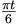) tells you the pattern it follows from there.

So, if you do 1.605 - 0.25 instead of the 1.1, it just affects where it starts on the "y-axis".

The point I was trying to make before is that W(t) should be seen as a "black box" - you can't change anything inside it.

Now, in your second point there you seem to be saying that we effectively have a reservoir which starts with 1.605-0.25 million gallons - however this isn't right since this level is at t=23, not at t=0 which is the true "start".

Although you might not understand exactly why, I hope you can see that the formula you are suggesting is wrong - if you try t=23 in it, you get a different value from what you expect.

### #13naav

• Gender:Male

Posted 04 October 2006 - 09:13 PM

Thank you very, very muchKindest Regards

#### 1 user(s) are reading this topic

0 members, 1 guests, 0 anonymous users